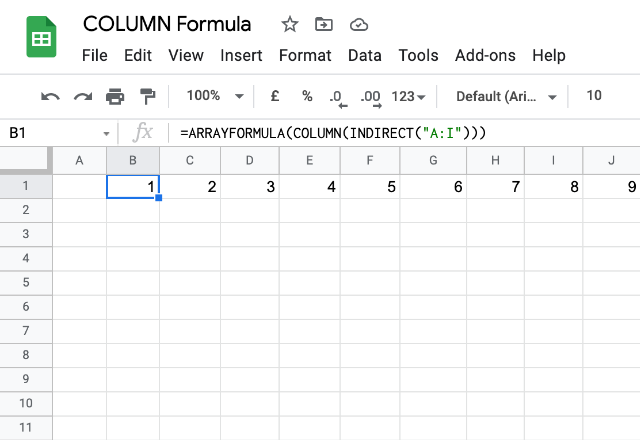# How to use the COLUMN formula in Google SheetsThe COLUMN formula is one of the lookup functions available within Google Sheets. It gives us the column number where the specified cell or a range of cells are located.

### Syntax

COLUMN([cell_reference])

cell_reference – is the address reference to the cell whose column number we need. This is an optional parameter. So, if not specified, the COLUMN formula considers the address of the cell where we keyed in the formula.

Please note that if the cell_reference parameter is not a single cell, but is a range of multiple cells, the formula returns only the column number of the first column within the cell_reference.

### Usage: COLUMN Formula

Let us get our understanding of the formula more concrete by means of a few examples. Please see the snapshot below.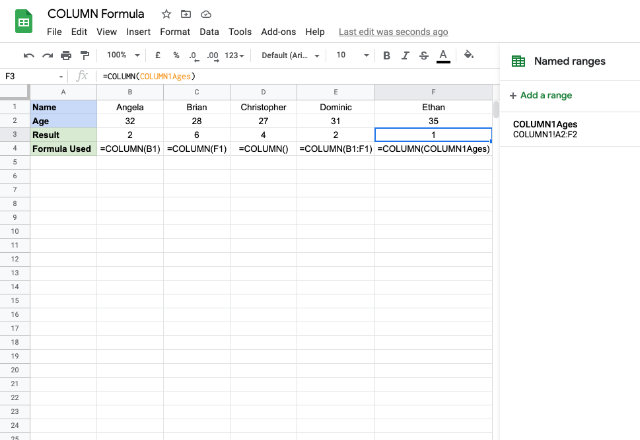The first example took B1 for cell_reference and accordingly returned 2 as output. Similarly, for F1, it returned 6 as output for the second example. The third case doesn’t have any input for the cell_reference, hence it returned the column number of the cell in which we keyed in the formula.

In the fourth example, we provided an address to a range of cells as opposed to a single cell. And, it returned the number of the first column within the range B1:F1, which is 2.

It is interesting to note that we provided a named range reference as input for the cell_reference parameter in the last example case. As shown on the extreme right of the above image, the “COLUMN1!Ages” range spans from A2 to F2. Therefore, the result is the first column number of range A1:F2.

##### Use case: Auto-filling numbers

One of the useful ways we can use the COLUMN formula is when we use it in conjunction with the ARRAYFORMULA. We use the following method to have Google Sheets fill up series of numbers for us without having to enter them ourselves.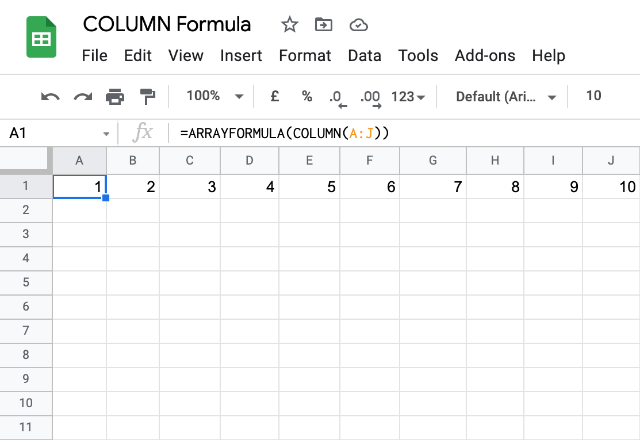This utility of the above formula is very handy indeed. Even if we try and insert a column anywhere between the existing columns, the numbering is taken care of automatically. To illustrate this, we will insert a column between columns F and G as shown below. And we will notice we don’t have to do anything to adjust the numbering – Google Sheets does that for us automatically.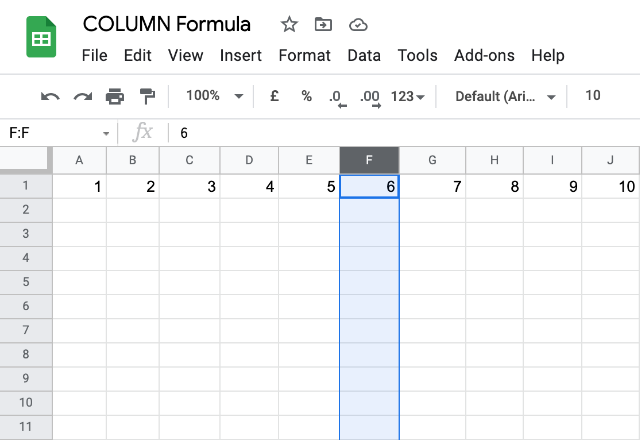But then, there is a small problem. What if we try inserting a column above the before cell A1? Doing so would shift the cell right and accordingly adjusts the formula as shown below, which is probably undesirable.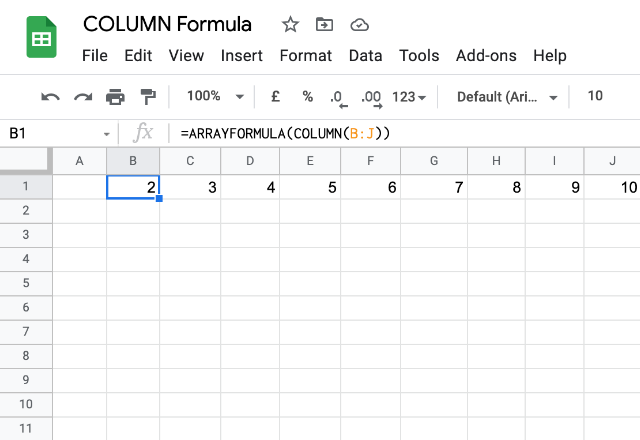We can fix this issue using the INDIRECT formula as shown below. Even if we insert a column before, it doesn’t affect the numbering. However, the address reference A:L has now become static. So, inserting columns between A through L will not adjust the reference to A:M anymore (like it did in the third snapshot).